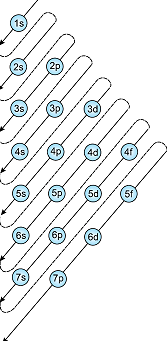# The incorrect order of energy of following orbitals is Option 1) 3s < 3p < 4s < 4d Option 2) 5p < 5d < 5f < 6p Option 3) 7s < 5f < 6d < 7p Option 4) 4d < 5p < 6s < 4f < 5d

As we learned in concept

Aufbau Principle -

In the ground state of the atoms, the orbitals are filled in order of their increasing energies.

- whereinThe order of energy of orbitals is determined by n+l where n= principal quantum number and l = azimuthal quantum number

So, 6p = 6+1 = 7

5f = 5+3 = 8

5d = 5+2 = 7

5p = 5+1 = 6

Clearly, to state that 5p<5d<5f<6p is incorrect.

Option 1)

3s < 3p < 4s < 4d

This option is incorrect.

Option 2)

5p < 5d < 5f < 6p

This option is correct.

Option 3)

7s < 5f < 6d < 7p

This option is incorrect.

Option 4)

4d < 5p < 6s < 4f < 5d

This option is incorrect.

### Preparation Products

##### Knockout BITSAT 2021

It is an exhaustive preparation module made exclusively for cracking BITSAT..

₹ 4999/- ₹ 2999/-